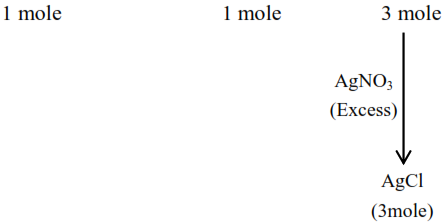# Three moles of

Question:

Three moles of $\mathrm{AgCl}$ get precipitated when one mole of an octahedral co-ordination compound with empirical formula $\mathrm{CrCl}_{3} .3 \mathrm{NH}_{3} .3 \mathrm{H}_{2} \mathrm{O}$ reacts with excess of silver nitrate. The number of chloride ions satisfying the secondary valency of the metal ion is

Solution:

Mole of $\mathrm{AgCl}$ precipitated is equal the mole of $\mathrm{Cl}^{-}$ present in ionization sphere.

$\left[\mathrm{Cr}\left(\mathrm{H}_{2} \mathrm{O}\right)_{3}\left(\mathrm{NH}_{3}\right)_{3}\right] \mathrm{Cl}_{3} \rightarrow\left[\mathrm{Cr}\left(\mathrm{H}_{2} \mathrm{O}\right)_{3}\left(\mathrm{NH}_{3}\right)_{3}\right]^{3+}+3 \mathrm{Cl}^{-}$Since none of $\mathrm{Cl}^{-}$is present in the co-ordination sphere. Therefore answer is zero.